Next: The force on a Up: Relativity and electromagnetism Previous: Fields due to a

Relativistic particle dynamics

Consider a particle which, in its instantaneous rest frame, has mass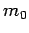and constant acceleration in the-direction. Let us transform to a frame, in the standard configuration with respect to, in which the particle's instantaneous velocity is. What is the value of, the particle's instantaneous-acceleration, in S?

The easiest way in which to answer this question is to consider the acceleration 4-vector [see Eq. (1429)]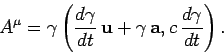(1541)

Using the standard transformation, (1397)-(1400), for 4-vectors, we obtain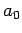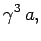(1542)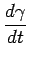(1543)

Equation (1542) can be written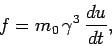(1544)

where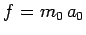is the constant force (in the-direction) acting on the particle in.

Equation (1544) is equivalent to(1545)

where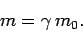(1546)

Thus, we can account for the ever decreasing acceleration of a particle subject to a constant force [see Eq. (1542)] by supposing that the inertial mass of the particle increases with its velocity according to the rule (1546). Henceforth,is termed the rest mass, andthe inertial mass.

The rate of increase of the particle's energysatisfies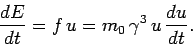(1547)

This equation can be written(1548)

which can be integrated to yield Einstein's famous formula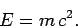(1549)

The 3-momentum of a particle is defined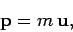(1550)

whereis its 3-velocity. Thus, by analogy with Eq. (1545), Newton's law of motion can be written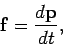(1551)

whereis the 3-force acting on the particle.

The 4-momentum of a particle is defined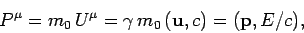(1552)

whereis its 4-velocity. The 4-force acting on the particle obeys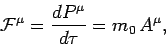(1553)

where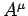is its 4-acceleration. It is easily demonstrated that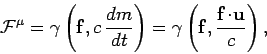(1554)

since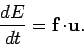(1555)Next: The force on a Up: Relativity and electromagnetism Previous: Fields due to a
Richard Fitzpatrick 2006-02-02